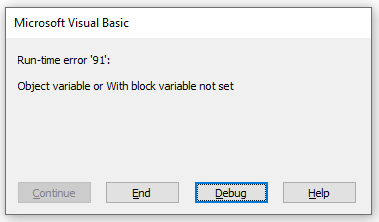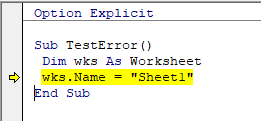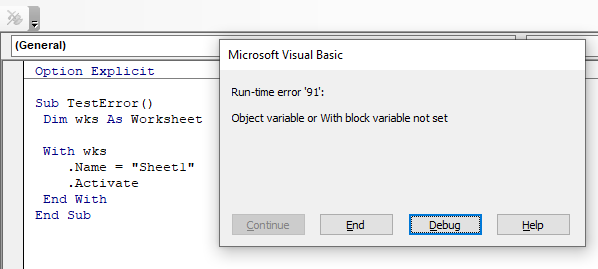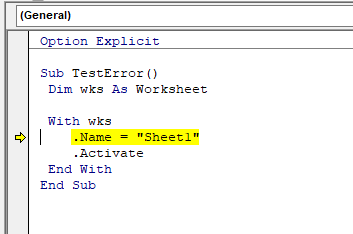# VBA Object Variable or With Block Variable Not Set Error

This article will explain the VBA object variable or with block variable not set error.This relatively common error occurs for exactly the reason that the message box says – the object variable or with block variable has not been set yet!

## Object Variable Not Set

Let us consider the following code:

``````Sub TestObject()
Dim wks as Worksheet
wks.Name = "Sheet1"
End Sub``````

We have declared a new object variable called “wks” as a Worksheet.

We are then attempting to name this sheet – Sheet1

However, when we run the code, we get the run-time error. When we click on the Debug button, the code stops at the line where we are trying to name the sheet.We are trying to populate the variable “wks” – but we haven’t actually allocated the variable to a specific sheet – we have only declared it as a variable. Therefore, although the variable is declared, the object doesn’t actually exist!

Let us consider the amended code below:

``````Sub TestObject()
Dim wks as Worksheet
Set wks = ActiveSheet
wks.Name = "Sheet1"
End Sub``````

We have now created the object with this line of code:

``Set wks = ActiveSheet``

The code will then run without error.

## With Block Variable Not Set

Let us now consider this code:

``````Sub TestWith()
Dim wks As Worksheet
With wks
.Name = "Sheet1"
.Activate
End With
End Sub``````

When we run this code, we get the same error:When we click on debug, the code stops within the WITH….END WITH block – hence the with block variable error.The error is actually the same and once again, by creating the object, we will solve the error.

``````Sub TestWith()
Dim wks As Worksheet
Set wks = ActiveSheet
With wks
.Name = "Sheet1"
.Activate
End With
End Sub``````